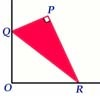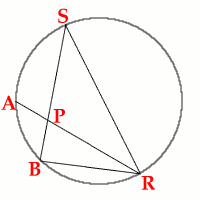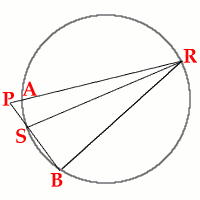#### You may also like### Baby Circle

A small circle fits between two touching circles so that all three circles touch each other and have a common tangent? What is the exact radius of the smallest circle?### Kissing

Two perpendicular lines are tangential to two identical circles that touch. What is the largest circle that can be placed in between the two lines and the two circles and how would you construct it?### Set Square

A triangle PQR, right angled at P, slides on a horizontal floor with Q and R in contact with perpendicular walls. What is the locus of P?

# Fixing It

##### Age 16 to 18Challenge Level$\mathbf{A}$ and $\mathbf{B}$ are fixed points on a circle, while $\mathbf{RS}$ is a variable diameter. $\mathbf{P}$ is the intersection of $\mathbf{AR}$ and $\mathbf{BS}$. We make the conjecture that the locus of $\mathbf{P}$ isa a second circle through the points $\mathbf{A}$ and $\mathbf{B}$.

Edward Wallace of Graveney School, Tooting sent a very good proof of this conjecture.

First consider the two cases illustrated in the given diagrams. It is impossible to have $\mathbf{R}$ and $\mathbf{S}$ both on the minor arc of the circle. The other two cases, where $\mathbf{R}$ and $\mathbf{S}$ exchange positions, and $\mathbf{P}$ is outside the original circle, are proved similarly.

Case 1

Both $\mathbf{R}$ and $\mathbf{S}$ are on the major arc $\mathbf{AB}$ so that $\mathbf{P}$ is inside the original circle.

Case 2

$\mathbf{S}$ is on the minor arc $\mathbf{AB}$ and $\mathbf{R}$ on the major arc so that $\mathbf{P}$ is outside the original circle.

In Case 1, $\angle\mathbf{ARB}$ is unchanging (invariant) as $\mathbf{R}$ moves on the circumference because angles subtended on the circumference of a circle by a fixed arc of the circle are equal. Hence ($\angle \mathbf{ARB} = m^o$ (constant). Also $\angle \mathbf{RBS}=90^o$ because the angle subtended by a diameter is a right angle.

It follows that $\angle \mathbf{RPB}=(90 - m)^o$ because the angles of the triangle $\mathbf{BRP}$ add up to $180^o$.

Hence $\angle \mathbf{APB}$ is constant and equal to $(90 + m)^o$ because angles on a line add up to $180^o$. This is a necessary and sufficient condition for P to lie on the circumference of a circle of which $\mathbf{AB}$ is a chord. Let us call this arc $\mathbf{AP_{1}B}$ to emphasise the fact that, in this case, $\mathbf{P_1}$ is inside the original circle.

In Case 2, exactly the same argument applies, giving $\angle \mathbf{ARB} = m^o$ (the same value as in Case 1) and $\angle\mathbf{RBS}=90^o$, but in this case $\angle\mathbf{APB}$ is the same angle as $\mathbf{RPB}$ so $\angle\mathbf{APB}=(90 - m)^o$ (again constant).

As before, this is a necessary and sufficient condition for $\mathbf{P}$ to lie on the circumference of a circle of which $\mathbf{AB}$ is a chord. We call this arc $\mathbf{AP_{2}B}$ to emphasise the fact that now $\mathbf{P_2}$ is outside the original circle.

Note that:
$\angle\mathbf{AP_{1}B} + \angle\mathbf{AP_{2}B} = (90 + m)^o + (90 - m)^o = 180^o$ and hence $\mathbf{AP_{1}BP_{2}}$ is a cyclic quadrilateral which confirms that the locus of $\mathbf{P}$ is a single circle (and not two arcs of different circles).